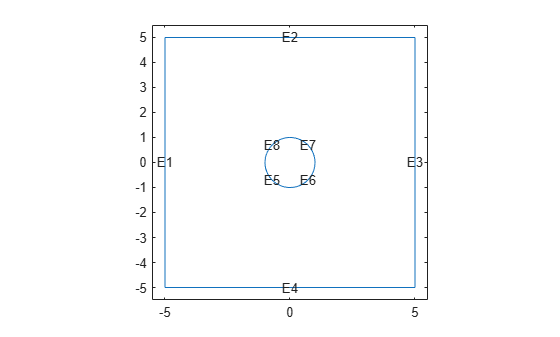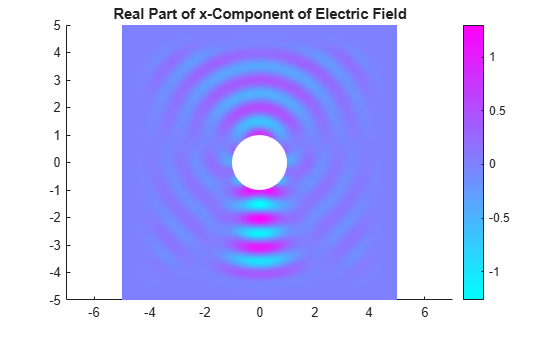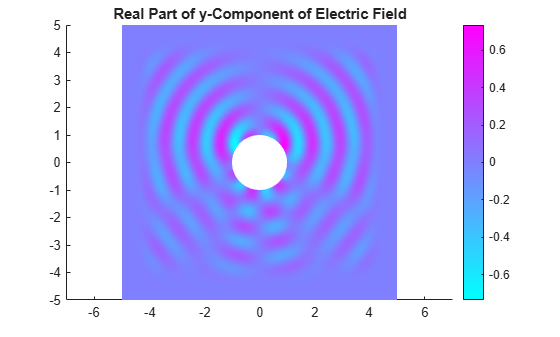# HarmonicResults

Harmonic electromagnetic solution

Since R2022a

## Description

A `HarmonicResults` object contains the electric or magnetic field, frequency, and mesh values in a form convenient for plotting and postprocessing.

The electric or magnetic field values are calculated at the nodes of the triangular or tetrahedral mesh generated by `generateMesh`. Electric field values at the nodes appear in the `ElectricField` property. Magnetic field values at the nodes appear in the `MagneticField` property.

To interpolate the electric or magnetic field to a custom grid, such as the one specified by `meshgrid`, use the `interpolateHarmonicField` function.

## Creation

Solve a harmonic electromagnetic analysis problem using the `solve` function. This function returns a solution as a `HarmonicResults` object.

## Properties

expand all

Electric field values at nodes, returned as an `FEStruct` object. The properties of this object contain the components of the electric field at nodes.

Magnetic field values at nodes, returned as an `FEStruct` object. The properties of this object contain the components of the magnetic field at nodes.

Solution frequencies, returned as a vector.

Data Types: `double`

Finite element mesh, returned as an `FEMesh` object. For details, see FEMesh Properties.

## Object Functions

 `interpolateHarmonicField` Interpolate electric or magnetic field in harmonic result at arbitrary spatial locations

## Examples

collapse all

For an electromagnetic harmonic analysis problem, find the x- and y-components of the electric field. Solve the problem on a domain consisting of a square with a circular hole.

Create an electromagnetic model for harmonic analysis.

`emagmodel = createpde("electromagnetic","harmonic");`

Define a circle in a square, place them in one matrix, and create a set formula that subtracts the circle from the square.

```SQ = [3,4,-5,-5,5,5,-5,5,5,-5]'; C = [1,0,0,1]'; C = [C;zeros(length(SQ) - length(C),1)]; gm = [SQ,C]; sf = 'SQ-C';```

Create the geometry.

```ns = char('SQ','C'); ns = ns'; g = decsg(gm,sf,ns);```

Include the geometry in the model and plot the geometry with the edge labels.

```geometryFromEdges(emagmodel,g); pdegplot(emagmodel,"EdgeLabels","on") xlim([-5.5 5.5]) ylim([-5.5 5.5])```Specify the vacuum permittivity and permeability values as 1.

```emagmodel.VacuumPermittivity = 1; emagmodel.VacuumPermeability = 1;```

Specify the relative permittivity, relative permeability, and conductivity of the material.

```electromagneticProperties(emagmodel,"RelativePermittivity",1, ... "RelativePermeability",1, ... "Conductivity",0);```

Apply the absorbing boundary condition with a thickness of 2 on the edges of the square. Use the default attenuation rate for the absorbing region.

```electromagneticBC(emagmodel,"Edge",1:4, ... "FarField","absorbing", ... "Thickness",2);```

Specify an electric field on the edges of the hole.

```E = @(location,state) [1;0]*exp(-1i*2*pi*location.y); electromagneticBC(emagmodel,"Edge",5:8,"ElectricField",E);```

Generate a mesh.

`generateMesh(emagmodel,"Hmax",1/2^3);`

Solve the model for a frequency of $2\pi$.

`result = solve(emagmodel,"Frequency",2*pi);`

Plot the real part of the x-component of the resulting electric field.

```figure pdeplot(emagmodel,"XYData",real(result.ElectricField.Ex)); title("Real Part of x-Component of Electric Field")```Plot the real part of the y-component of the resulting electric field.

```figure pdeplot(emagmodel,"XYData",real(result.ElectricField.Ey)); title("Real Part of y-Component of Electric Field")```## Version History

Introduced in R2022a